# How to Get net working days in Excel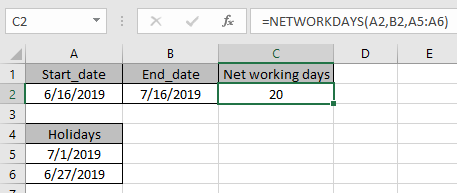In this article, we will learn How to Get net working days in Excel.

What are working and non working days ?

Usually Saturdays and Sundays are weekends meaning non working days. Sometimes calculating the total work days between two dates,  we can manually calculate the number of days between the days and subtract the number of days. This doesn't look easy and can lead to mistakes if the two given dates are too much apart. So for this we usually use NETWORKDAYS Function in Excel. NETWORKDAYS function has the fixed type of weekend which is Saturday and Sunday. So now we learn the extended version of NETWORKDAYS function which lets you edit the weekends as per choice.

NETWORKDAYS Function in Excel

To get the networking days between dates having holidays use NETWORKDAYS function in Excel. It takes Saturday and Sunday as default holidays.

NETWORKDAYS Function Syntax:

 =NETWORKDAYS(start_date, end_date, [holidays])

Start_date : count of first date

End_date : count of end date

Holidays : [optional] argument in the function

NOTE : The NETWORKDAYS Function includes both start date and end date in the count of networking days.

Example :

All of these might be confusing to understand. Let's understand how to use the function using an example. Here we have some sample dates where we need to find the total working days between the two given dates where Saturday and Sunday are non working days and considering national holidays as working days. Here we have start_date, end date and a couple of extra holidays.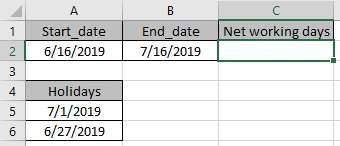Use the formula:

 =NETWORKDAYS(A2,B2,A5:A6)As you can see net working days are 20 in between dates having holidays.

NETWORKDAYS.INTL function in Excel

Here we have some sample dates where we need to find the total working days between the two given dates where Saturday and Sunday are non working days and considering national holidays as working days.

Use the formula:

 =NETWORKDAYS.INTL(B4, C4)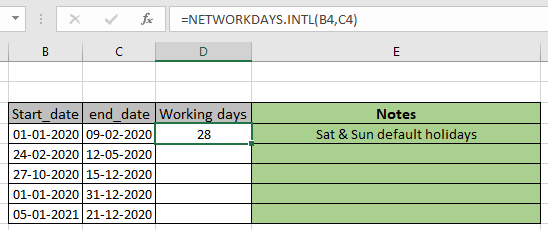As you see there are 28 working days between the dates taking Sat & Sun as default non working days.

Now we want to take the only Sunday as non working day in the week and considering national holidays as non working days. Here national holidays are called as holidays using named range.

Use the formula:

 =NETWORKDAYS.INTL(B5, C5, 11, holidays)

11 : argument for the Sunday as only non working days in a week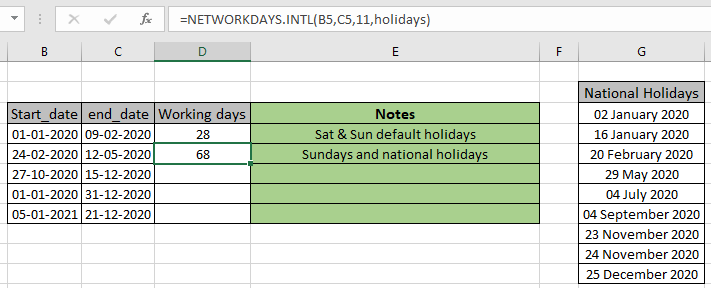As you can see the formula returns 68 days. This formula is very useful for the government employees as per conditions explained.

Masking days

Now an example is explained using the masking days in week. Considering all working days in a week and all national holidays as working.

Use the formula:

 =NETWORKDAYS.INTL(B6, C6, "0000000")

1 means non workday and 0 means workdayAs you can see, there are 50 working days between the two dates. Similarly you can find the working days between any two given dates. Below are some more examples.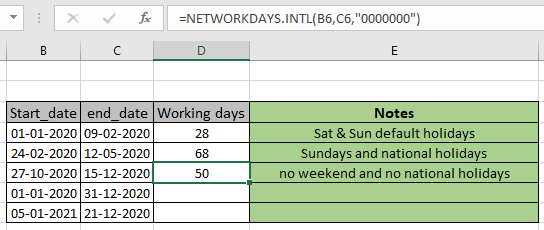In the D7 cell, Tuesday, Thursday, Saturday and Sunday are taken as non work days. But in the D8 cell you must be curious to know how the number of days can be negative. Yes you are right, days cannot be negative. The negative sign denotes that the end_date and start date are not in correct order whereas the magnitude 11 denotes the number of working days between the two dates.

Here are all the observational notes using the NETWORKDAYS function in Excel
Notes :

1. Excel stores dates as serial numbers and is used in calculation by the function. So it’s recommended to use dates as cell reference or using the DATE function instead of giving direct argument to the function. Check the validation of date values if the function returns #VALUE! Error.
2. If the start date is greater than the end date, the function returns the number of days with a negative sign.
3. If the start date and end date is the same date and that date is working, the function returns 1.
4.  If the start date or end date is out of range, then function returns #NUM! Error.
5. Holidays argument must be given as array reference, not the individual dates separated using commas, the function throws too many arguments error.

Hope this article about How to Get net working days in Excel is explanatory. Find more articles on calculating values and related Excel formulas here. If you liked our blogs, share it with your friends on Facebook. And also you can follow us on Twitter and Facebook. We would love to hear from you, do let us know how we can improve, complement or innovate our work and make it better for you. Write to us at info@exceltip.com.

Related Articles :

How to use the NETWORKDAYS function in Excel : Returns the working days between the two given dates using the NETWORKDAYS function.

How to use the WORKDAYS function in Excel : Returns a workday date value after days added to the start_date using the WORKDAYS function in Excel.

Count holidays between dates in Excel : count non working days between the two given dates using the DATEDIF and NETWORKDAYS function in Excel.

Extract days between dates ignoring years in Excel : count the days between the two dates using different functions and mathematical operation in Excel

Count Birth Dates By Month in Excel : count the number of dates lying in a particular month using the SUMPRODUCT and MONTH function in Excel.

SUM price by weekdays in Excel : sum price values corresponding to the number of dates in a particular weekday using the SUMPRODUCT and WEEKDAY function in Excel.

Popular Articles :

How to use the IF Function in Excel : The IF statement in Excel checks the condition and returns a specific value if the condition is TRUE or returns another specific value if FALSE.

How to use the VLOOKUP Function in Excel : This is one of the most used and popular functions of excel that is used to lookup value from different ranges and sheets.

How to use the SUMIF Function in Excel : This is another dashboard essential function. This helps you sum up values on specific conditions.

How to use the COUNTIF Function in Excel : Count values with conditions using this amazing function. You don't need to filter your data to count specific values. Countif function is essential to prepare your dashboard.

Terms and Conditions of use

The applications/code on this site are distributed as is and without warranties or liability. In no event shall the owner of the copyrights, or the authors of the applications/code be liable for any loss of profit, any problems or any damage resulting from the use or evaluation of the applications/code.